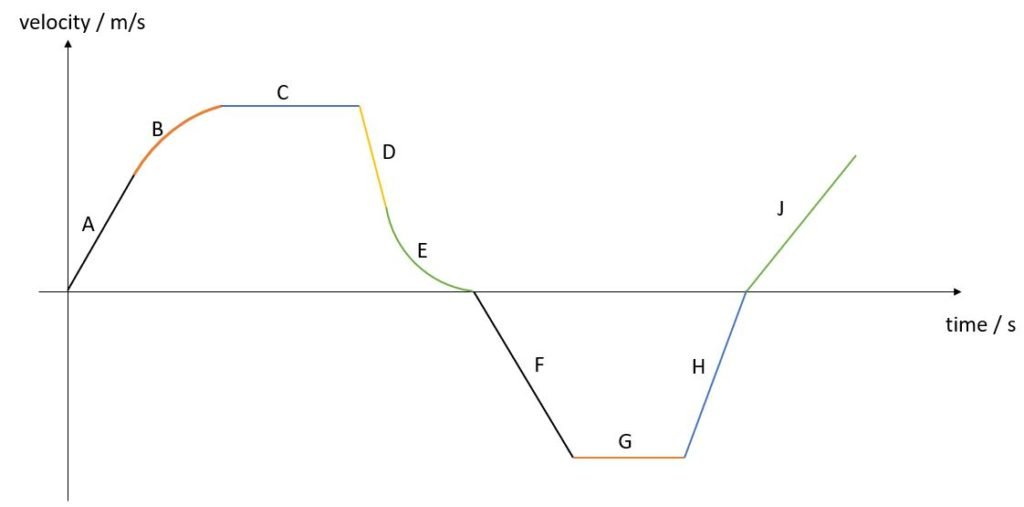# [Keywords] Kinematics

### Important Facts That You Must Know First!

1. Free fall is when an object experiences ONLY gravitational force and NO other forces.
2. Object can moves with constant speed but changing velocity. An example of such scenario is when an object is moving in a circular motion.
3. It is not always true that acceleration must be zero if an object is at rest. Object can have zero velocity (at rest) but non-zero acceleration. Famous example is when you throw an object upwards, it is momentarily at rest at the highest point but its acceleration is definitely non-zero there. For free fall, that acceleration will be equal to g=10m/s2. Do you know what will be the acceleration of a tennis ball (mid-way up) thrown upwards in free fall?
4. Whenever an object reaches a constant speed, can we call that terminal velocity? Although there is no formal definition, it is commonly agreed that terminal velocity referred to a maximum velocity attainable by an object in its fall in fluids. So for horizontal motion when a car experiences zero resultant force, we will say that the car reaches a constant speed rather than terminal velocity.
5. diva without i = dva. d –> v –> a. A useful abbreviation to remember when dealing with graph. d represents displacement/distance – time graph. v represents velocity/speed – time graph and a is acceleration – time graph. How to use this? Gradient of (d) –> v. Gradient of (v) –> a.

### Commonly Seen Questions

• Describe the motion of an object based on this velocity-time graph.Positive gradient = acceleration. Negative gradient = deceleration. However, we don’t follow this rule strictly in order to put thing into perspective. You will see this when we describe the motion for F and H.

A : object is undergoing constant acceleration.

B: object is undergoing decreasing acceleration.

C: object is undergoing constant velocity (zero acceleration).

D: object is undergoing constant deceleration.

E: object is undergoing decreasing deceleration and eventually comes to rest.

F: object is undergoing constant acceleration in opposite direction (a.k.a deceleration).

G: object is undergoing constant velocity and still moving in opposite direction.

H: object is undergoing constant deceleration and still moving in opposite direction (a.k.a acceleration) and eventually comes to rest.

J: object turns back and is undergoing constant acceleration.

NOTE: If the velocity of the object is known, a better answer will include that as well.

For example, for segment A, we can say that the object is undergoing constant acceleration where its velocity increases uniformly from 0 m/s to 10 m/s. Segment B, the object is undergoing decreasing acceleration where its velocity increases from 10 m/s to 15 m/s at a decreasing rate.

NOTE:

• If you are given displacement/distance – time graph, when describing the motion, you should include the velocity/speed and how displacement/distance changes. Basically, you describe the change in the value of the displacement/distance and also the gradient of the graph.
• Using the same understanding, if we are given a velocity/speed – time graph, we should describe the change in velocity/speed and also the acceleration which is the gradient of the graph.

• A parachutist jumps off from a height and reaches a terminal velocity after 5 seconds. Explain, in terms of forces acting on the parachutist, why he reaches terminal velocity.
• Initially, when he first just jumps off, only the weight of the parachutist is acting on him downward. As his speed increases, the air resistance acting on him also increases. This results in a decreasing downward resultant force acting on the parachutist. Since his mass remains constant, the parachutist is undergoing a decreasing acceleration. Eventually, when the magnitude of the air resistance equals to the magnitude of the weight, the resultant force is zero and hence the parachutist’s acceleration is zero. He reaches terminal velocity.